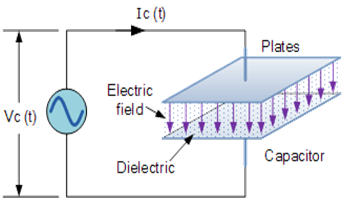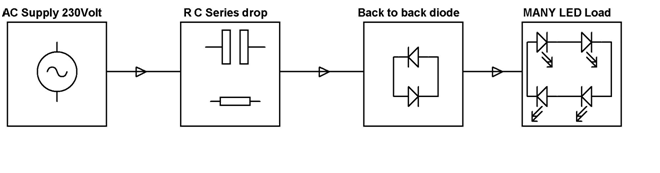# Mains Operated LED

### Introduction to LED

An LED or Light Emitting Diode is a simple PN junction diode, made of material with larger energy barrier. As the supply is given to the LED junction, the electrons move from the valence band to the conduction band. When the electron looses energy and falls back to its original state, a photon is emitted. This emitted light is in the frequency band of the visible frequency range of light.

This simple diode emits light when its p-n junction is biased by a voltage as low as 1 volt. Most of the LEDs work between 1.5 volts to 2 volts, but the high bright types especially the White, Blue and Pink LEDs require 3 volts to give maximum brightness. The current through the LED should be limited to 20 -30 milli amperes otherwise the device will be burned. White and Blue LEDs can tolerate up to 40 milli amperes current.

The LED has a semiconductor chip made up of Gallium compound which has the property of photon emission by the influence of current. The chip is connected to two terminal posts for providing supply voltage. The whole assembly is encapsulated in an epoxy case with the terminals projecting out. The long lead of the LED is positive while the short lead is negative. Originally, the semiconductor used in the LED was Gallium Arsenide Phosphate (GaAsP) while Gallium Aluminium Aeresnide (GaAlAs) is used now a days in high bright LEDs. Blue and White LEDs use Indium Gallium Nitride (InGaN) while the Multicolour LEDs use different combinations of materials to produce different colours. The White LED contains a blue chip with white inorganic phosphor. When the blue light strikes the phosphor, white light will be emitted.

LEDs emit light based on the Electroluminescence. The semiconductor material in the LED has both P type and N type regions. The p region carries positive charge carries called Holes while the N region releases electrons. The photon emitting material is sandwiched between the P and N layers. When a potential difference is applied between the P and N layers, the electrons from N layer move towards the active material and combines with holes. This releases energy in the form of light from the active material. Based on the type of active material, different colours will be produced.

### 8 types of LEDs and the material used in them

1. Aluminum Gallium Arsenide – Infrared LED

2. Aluminum Gallium Arsenide, Gallium Arsenide Phosphide, Gallium Phosphide – Red LED

3. Aluminum Gallium Phosphide, Gallium Nitride – Green LED

4. Aluminum Gallium Phosphide, Gallium Arsenide Phosphide, Gallium Phosphide – Yellow LED

5. Aluminium Gallium Indium Phosphide – Orange LED

6. Indium Gallium Nitride, Silicon Carbide, Sapphire, Zinc Selenide – Blue LED

7. Gallium Nitride based Indium Gallium Nitride – White LED

8. Indium Gallium Nitride, Aluminium Gallium Nitride – Ultraviolet LED

### 8 LED parameters

1. Luminous Flux- It is the amount of energy from the LED and is measured in Lumen(lm) or Milli lumen(mlm)

2. Luminous Intensity – It is the Luminous Flux covering an area and is measured in terms of Candela (cd). Brightness of LED depends on the luminous intensity.

3. Luminous Efficacy – It indicates the light in relation to the voltage applied. Its unit is lumen per watt (lm w).

4. Forward voltage (Vf) – It is the voltage drop across the LED. It ranges from 1.8 volts in Red LED to 2.2 volts in green and Yellow LEDs. In Blue and White LEDs, it is 3.2 volts.

5. Forward current (If) – It is the maximum current allowable through the LED. It ranges from 10 mA to 20mA in ordinary LED while 20mA to 40 mA in White and Blue LEDs. The high bright 1 watt LEDs requires 100 – 350 milli amperes current.

6. Viewing angle – It is also called Off- axis angle. It is the luminous intensity fall to half axis value. This results in full brightness in full on condition. The high bright type LEDSs have narrow viewing angle so that the light will be focused into a beam.

7. Energy level – The energy level in the light output depends on the voltage applied and the charge in the electrons of the semiconductor. The energy level is E=qV where q is the charge in the electrons and V is the applied voltage. q is typically -1.6×1019 Joule.

8. Wattage of LED – It is the forward voltage multiplied by the forward current. If excess current is flowing through the LED, its life will be reduced. So a series resistor, typically 470 ohms to 1K is used to limit current through the LED.

The LED resistor can be selected using the formula Vs – Vf / If. Where Vs is the input voltage, Vf is the forward voltage of LED and If is the forward current of LED.

### Need for AC supply for driving LED

For applications involving low power such as in mobile phones, it is possible to use dc supply for an LED. However for large scale applications like Traffic Lights using LEDs, it is actually inconvenient to use DC. This is because as distance increases DC power transmission contributes to more losses and also it is quite inexpensive to use devices for DC-DC conversion. As a result it is more suitable to use AC supply for high end application like glowing of large number of LEDs.

### Capacitor as an AC voltage limiterThe capacitor has a property of opposing the change in applied voltage by drawing or supplying current from the circuit, as they charge or discharge. The current across the capacitor is given as

I = CdV/dt

Where C is capacitance, dV/dt denotes change in voltage. I is the charge between the plates per unit time or the current.

The current through a capacitor is a reaction against the change in voltage. Therefore for a high instantaneous voltage, the current is zero. In other words voltage lags the current by 90 degrees. This property of the capacitor makes it usable as a voltage reducer for AC power supply. However this depends on the capacitance value and the frequency. Higher the frequency and the capacitance, lesser is the reactance.

### Application involving use of AC mains to drive LEDLED or Light Emitting Diodes can be directly operated through AC mains supply simply by using the combination of a capacitor and a resistor. The AC main supply of 220V is converted to low voltage AC using a transformer.  The capacitor is used as a voltage limiter where as the resistor is the current limiter. The diodes with high PIV(1000V) are used to protect the LEDs from high voltage.

Normally the voltage drop across a white led is about 1.5V. The LEDs are connected in two series-parallel combinations. If 12 LEDs are used in each combination, the voltage drop across the LED combination is around 30V. The resistor acts as a current limiter and provides a voltage drop of approximately 30V. Thus with the combination of a capacitor and the resistor, it is possible to drive a series of LEDs.  The value of the resistor depends on the number of LEDs used. Since the LED rating is at 15mA, the current through each LED will be 15mA and the total current through the two set of LED combination will be 30mA, causing a voltage drop of 30V across the 1k resistor.

I hope you have got an idea about the concept of mains operated LED if any furthermore quires on this topic or on the concept of the electrical and electronic projects leave the comments section below.